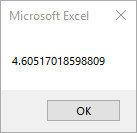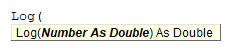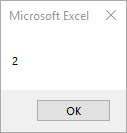# VBA Log Function

## Log Description

Returns the natural logarithm of a number.

## Simple Log Examples

``````Sub Abs_Example()
MsgBox Log(100)
End Sub
``````

This code will return 4.60517018598809## Log Syntax

In the VBA Editor, you can type  “Log(” to see the syntax for the Log Function:The Log function contains an argument:

Number: Any valid numeric expression greater than zero.

## Examples of Excel VBA Log Function

``MsgBox Log(10)``

Result: 2.30258509299405

``MsgBox Log(32)``

Result: 3.46573590279973

All the above examples are cases with the base e(the base of the natural logarithm).

To calculate the logarithm with any base, you can use the code as following function.

``````Function LogAny(b As Double, x As Double) As Double
' b: base x: a given number
LogAny = Log(x) / Log(b)
End Function

Sub Log_Example1()
MsgBox LogAny(10, 100)
End Sub``````

The result will be as following.Stop searching for VBA code online. Learn more about AutoMacro - A VBA Code Builder that allows beginners to code procedures from scratch with minimal coding knowledge and with many time-saving features for all users!